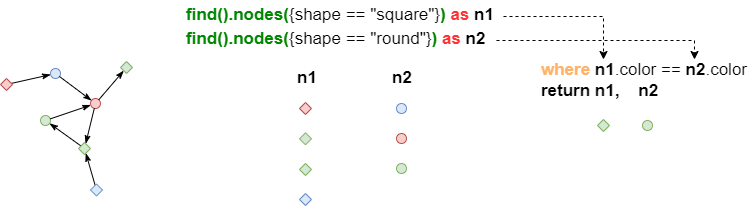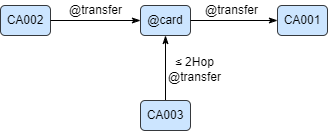# Change Nickname

Current Nickname:

Certifications

Certificate Issued at Valid until Serial No. File
Serial No. Valid until

Not having one? Apply now! >>>

Invoice

ProductName CreateTime ID Price File
ProductName CreateTime ID Price File

# WHERE | Check Row by Row

## Format, Parameters

WHERE can keep the rows of data in the data streams that meet the conditions, and discard those that do not meet the conditions.

Syntax:

• Format 1: where `<condition>`
• Format 2: where `<query>` (Under development)
• Parameters: see table below
• Affected columns: all aliases and homologous columns from `<condition>` and `<query>`; non-homologous aliases will be trimmed to the shortest length so that they can become homologous columns before calculation
Name Category Specification Description
`<condition>` filter Filter with curly brackets removed Judgement conditions, rows that are judged as TRUE will be kept
`<query>` query / Query statement, rows that return results will be kept

WHERE can seperate some filtering conditions from Ultipa Filter to improve UQL readability; it can also realize subgraph queries as complex as `graph()` command.

## Analysis

``````find().nodes({shape == "square"}) as n1
find().nodes({shape == "round"}) as n2
where n1.color == n2.color
return n1, n2
``````

In the UQL statement above, WHERE trims the two non-homologous columns outputted from 2 node queries to the shortest length，3 rows, then filter each row## where `<condition>`

Example: find three-step transfer paths from Card CA001 to Card CA002, with two intermittent cards in the paths containing at least one card whose level is 5

``````n({_id == "CA001"}).e().n({@card} as n1)
.e().n({@card} as n2)
.e().n({_id == "CA002"}) as p
where n1.level == 5 || n2.level == 5
return p{*}
``````

## where `<query>` (Under Development)

Example: find an intermittent card named "agent" that satisfies conditions as shown in the image below: Card CA002 transfers money to Card CA001 via agent; agent is a neighbor to Card CA003 within 2 hops``````n({_id == "CA002"}).re().n({@card} as agent).re().n({_id == "CA001"})
where n(agent).e()[*:2].n({_id == "CA003"})
return agent{*}
``````

Analysis: WHERE further filters data columns "agent": it judges if the shortest paths from "agent" to Card CA003 exist, if true, then it passes "agent" to later "return"

The example above can be put in a subgraph template as shown below:

``````graph([
n({_id == "CA002"}).re().n({@card} as agent).re().n({_id == "CA001"}),
n(agent).e()[*:2].n({_id == "CA003"})
])
return agent{*}
``````